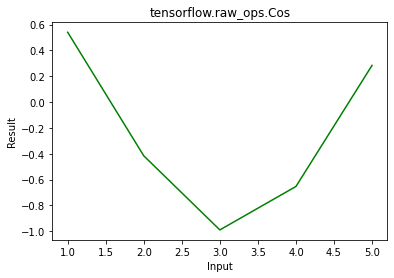# Python – tensorflow.raw_ops.Cos()

TensorFlow is open-source python library designed by Google to develop Machine Learning models and deep learning  neural networks. TensorFlow raw_ops provides low level access to all TensorFlow operations. Cos() is used to find element wise cosine of x.

Syntax: tf.raw_ops.Cos(x, name)

Parameters:

• x: It’s the input tensor. Allowed dtype for this tensor are bfloat16, half, float32, float64.
• name(optional): It defines the name for the operation.

Returns:  It returns a tensor of same dtype as x.

Note: It only takes keyword arguments.

Example 1:

## Python3

 `# Importing the library` `import` `tensorflow as tf`   `# Initializing the input tensor` `a ``=` `tf.constant([``1``, ``2``, ``3``, ``4``, ``5``], dtype ``=` `tf.float64)`   `# Printing the input tensor` `print``(``'Input: '``, a)`   `# Calculating cosine` `res ``=` `tf.raw_ops.Cos(x ``=` `a)`   `# Printing the result` `print``(``'Result: '``, res)`

Output:

```Input:  tf.Tensor([1. 2. 3. 4. 5.], shape=(5, ), dtype=float64)
Result:  tf.Tensor([ 0.54030231 -0.41614684 -0.9899925  -0.65364362  0.28366219], shape=(5, ), dtype=float64)```

Example 2: Visualization

## Python3

 `# importing the library` `import` `tensorflow as tf` `import` `matplotlib.pyplot as plt`   `# Initializing the input tensor` `a ``=` `tf.constant([``1``, ``2``, ``3``, ``4``, ``5``], dtype ``=` `tf.float64)`   `# Calculating cosine` `res ``=` `tf.raw_ops.Cos(x ``=` `a)`   `# Plotting the graph` `plt.plot(a, res, color ``=``'green'``)` `plt.title(``'tensorflow.raw_ops.Cos'``)` `plt.xlabel(``'Input'``)` `plt.ylabel(``'Result'``)` `plt.show()`

Output:Whether you're preparing for your first job interview or aiming to upskill in this ever-evolving tech landscape, GeeksforGeeks Courses are your key to success. We provide top-quality content at affordable prices, all geared towards accelerating your growth in a time-bound manner. Join the millions we've already empowered, and we're here to do the same for you. Don't miss out - check it out now!